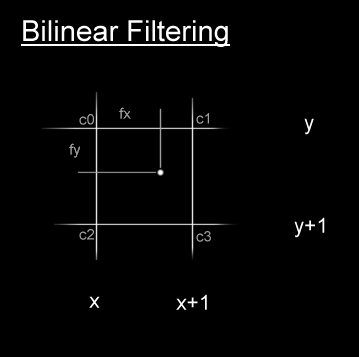The Art of Demomaking - Issue 06 - Bitmap Distortion by (27 September 1999) Return to The Archives
 IntroductionI won't waste time with whitty introductions this week since we have a lot to learn ;) So lets get down to business...In this tutorial you will find out how simple bitmap distortion is, and how you can drastically increase visual quality by performing bilinear filtering. I will also tell you about cache efficiency.

 DistortionSo far for every pixel we have drawn, we used information from a corresponding index in another buffer. The idea behind distortion is that we use a two dimensional displacement to access the other buffers.

The easiest effect that uses displacement simply involves copying a pixel from a displaced location: distortion.

 ``` offset = 0; for (j=0; j

Just like for the plasma, we can precalculate much more if the two displacement functions remain constant over time. Again, we simply translate the functions instead. So two extra buffers are needed to store the two displacement functions.

Note that no range checking is performed, so if any of the displaced coordinates x or y are too big, the image will wrap around. The program may even hang. This can be fixed by either adding clipping, or precalculating both displacement functions carefully.

 Bilinear FilteringNow you may notice our variables x and y in the algorithm above are both integers. This means that any distortion we introduce is rounded to the nearest pixel. This can produce some disappointing results when the distortion is close to zero. A solution is to introduce bilinear filtering. You've probably all heard of bilinear filtering for textures applied to 3D polygons. This is really quick when done in hardware, but our aim will be to make sure we can get it quick in software.

Here's the theory behind bilinear filtering. Say we have the coordinates of a texel (pixel from a texture) available in floating point. We could just round those values, and load the nearest texel. That would be quick, but not as accurate as it could be. What we do is find the 4 closest texels, and linearly interpolate their colour values in both directions. And voila, we get bilinear filtering.This is what the equation to get the bilinear filtered value looks like. A few simplifications are possible, but i'll leave that to you.

 ``` x and y are the integer part of the texel coordinates fx and fy are the fractional part c0 is the texel at (x,y) c1 is the texel at (x+1,y) c2 is the texel at (x,y+1) c3 is the texel at (x+1,y+1) c = c0 * (1-fx) * (1-fy) + c1 * fx * (1-fy) + c2 * (1-fx) * fy + c3 * fx * fy ```

The output quality is greatly enhanced by bilinear filtering. When you get used to it, it's hard to do without it. But the obvious major draw back is speed. We have to load 4 times more bytes from memory, and multiply them together. Even with low precision fixed point, the demo is about twice slower with bilinear filtering... which is acceptable I suppose.

 The Down SideAs you may have noticed, the computation required to distort an image (even without bilinear filtering) is slightly higher than just copying. An extra multiplication and a couple of additions per pixel are needed. But the speed lost in processing is however insignificant compared with the draw back of not addressing the memory in consecutive order. I was going to talk about cache at a later date, but since there's not much more to say about distortion, I'll cover that topic now.

 The CacheFinal words